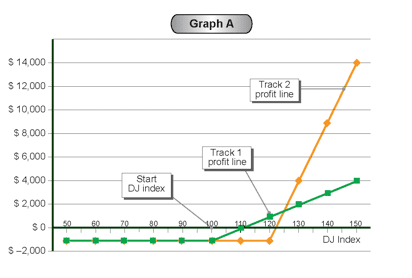# Strategy Number 1 – Long Call Options

 Market Data DJ Index 100 points Prices Call 100 \$1,000

Strategy name:

Long Call option.

Recommended use of strategy

Expectation of increase in DJ Index.

Strategy components

Example: Purchasing Call 100 at a price of \$1,000

Expenses / Income from building the strategy (at start date)

Expenditure of \$1,000.

Note: In the examples in this book, we use “convenient numbers” such as DJ Index 100 and option price of \$1,000. This is in order to simplify the discussion. In reality, the numbers are of course different.

Strategy graph:In this example, if the DJ Index is at 150 points (point A), the profit will be \$4,000.

If the index is at 100 (point B) the loss will be \$1,000 and if the index is at, 110 (point C), the profit will be \$0, this being the break-even point.

As previously mentioned, when we are in the loss area, the profit line is under the “0” line. When we are in the profit area, the profit line is above the “0” line.

As you can see, the profits from buying a Call option are unlimited, while the loss is limited to \$1,000 (the cost of the option).

When the DJ Index is below 100 points, the purchaser will not exercise the option.

Caution:

Despite the “limited loss” (in this case \$1,000), this represents the whole of the amount invested.

Auxiliary table for building the profit line

 DJ  Index (Horizontal axis) (Fixed expense)/fixed income Variable expenses Variable income (Call contribution) Total profit/(loss)(Vertical axis )2+3+4 1 2 3 4 5 80 (\$1,000) —– —– (\$1,000) 90 (\$1,000) —– —– (\$1,000) 100 (B) (\$1,000) —– —– (\$1,000) 110 (C) Breakeven point (\$1,000) —– \$1,000 \$0 120 (\$1,000) —– \$2,000 \$1,000 130 (\$1,000) —– \$3,000 \$2,000 140 (\$1,000) —– \$4,000 \$3,000 150 (A) (\$1,000) —– \$5,000 \$4,000

Explanation of table:

Column 1 – The DJ Index in 10-point intervals

Column 2 – There is a fixed expense of \$1,000 resulting from the purchase of the option. (The figure in brackets denotes an expense)

Column 3 – There are no variable expenses for this strategy.

Column 4 – This income results from exercising the option. For example, when the
index is 130, the profit from the option is \$3,000, according to the
following calculation:       \$13,000 (DJ Index X \$100)

\$10,000 (Strike price X \$100)

Profit:        \$3,000

Column 5 – Obtained by totaling columns 2+3+4